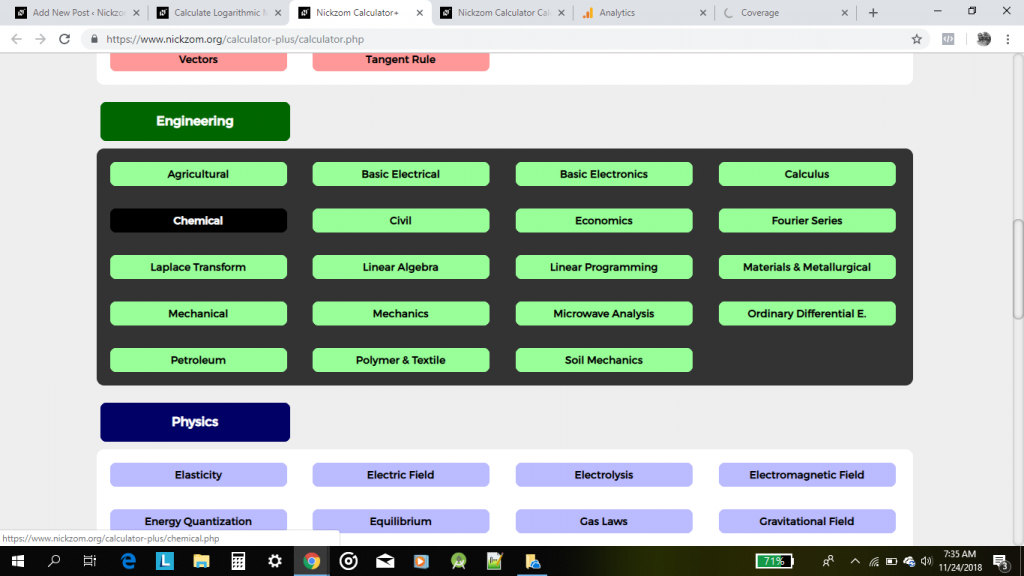## Nickzom Calculator Calculates the Logarithmic Mean Radius in Heat Transfer of Chemical Engineering

Heat Transfer is one of the major courses every Chemical Engineering student or professional faces at one point in life. There are various sections of heat transfer but for the purpose of this post. Our primary focus is to show you how Nickzom Calculator solves Logarithmic Mean Radius.

To get the answer for Logarithmic Mean Radius in Heat Transfer is very simple and one requires only two parameters which are:

• Outside Radius of the Cylinder
• Inside Radius of the Cylinder

Without Further Ado, let me proceed, click on the Calculator Map on Nickzom Calculator+ (Professional Version) – The Calculator EncyclopediaClicking on Chemical under Engineering, displays 3 major topics that can be handled by Nickzom Calculator in Chemical Engineering which are:

• Fluid Mechanics
• Heat Transfer
• Mass Transfer